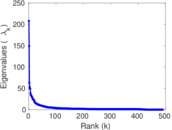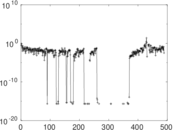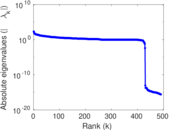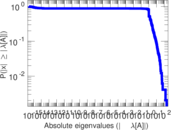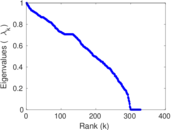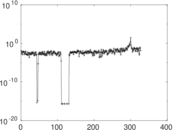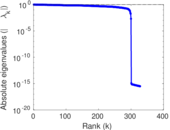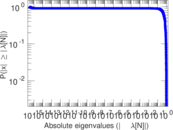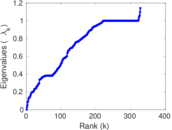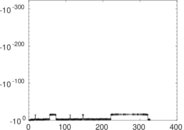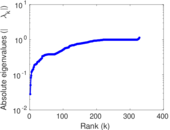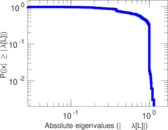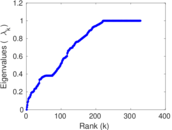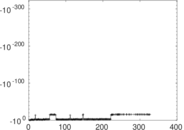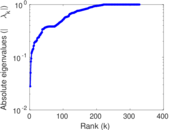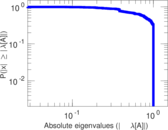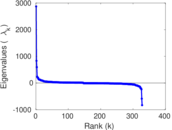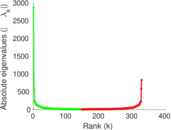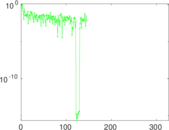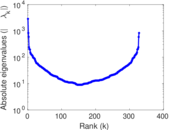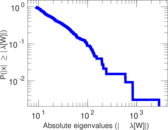# Wikiversity edits (ja)

This is the bipartite edit network of the Japanese Wikiversity. It contains users and pages from the Japanese Wikiversity, connected by edit events. Each edge represents an edit. The dataset includes the timestamp of each edit.

 Code `yja` Internal name `edit-jawikiversity` Name Wikiversity edits (ja) Data source http://dumps.wikimedia.org/ AvailabilityDataset is available for download Consistency checkDataset passed all tests Category Authorship network Dataset timestamp 2017-10-20 Node meaning User, article Edge meaning Edit Network formatBipartite, undirected Edge typeUnweighted, multiple edges Temporal dataEdges are annotated with timestamps

## Statistics

 Size n = 2,888 Left size n1 = 492 Right size n2 = 2,396 Volume m = 8,599 Unique edge count m̿ = 4,769 Wedge count s = 523,224 Claw count z = 67,627,959 Cross count x = 7,572,592,760 Square count q = 128,525 4-Tour count T4 = 3,130,762 Maximum degree dmax = 824 Maximum left degree d1max = 824 Maximum right degree d2max = 481 Average degree d = 5.954 99 Average left degree d1 = 17.477 6 Average right degree d2 = 3.588 90 Fill p = 0.004 045 53 Average edge multiplicity m̃ = 1.803 10 Size of LCC N = 2,521 Diameter δ = 12 50-Percentile effective diameter δ0.5 = 3.497 19 90-Percentile effective diameter δ0.9 = 5.260 51 Median distance δM = 4 Mean distance δm = 4.032 04 Gini coefficient G = 0.740 542 Balanced inequality ratio P = 0.207 001 Left balanced inequality ratio P1 = 0.133 736 Right balanced inequality ratio P2 = 0.286 080 Relative edge distribution entropy Her = 0.814 715 Power law exponent γ = 2.932 07 Tail power law exponent γt = 2.491 00 Tail power law exponent with p γ3 = 2.491 00 p-value p = 0.005 000 00 Left tail power law exponent with p γ3,1 = 1.821 00 Left p-value p1 = 0.250 000 Right tail power law exponent with p γ3,2 = 3.291 00 Right p-value p2 = 0.640 000 Degree assortativity ρ = −0.151 704 Degree assortativity p-value pρ = 5.980 47 × 10−26 Spectral norm α = 208.036 Algebraic connectivity a = 0.028 020 1 Spectral separation |λ1[A] / λ2[A]| = 1.397 43 Controllability C = 2,016 Relative controllability Cr = 0.701 950

## Plots

### Fruchterman–Reingold graph drawing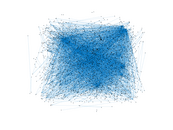### Degree distribution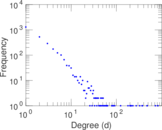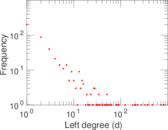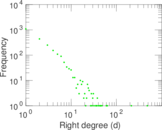### Cumulative degree distribution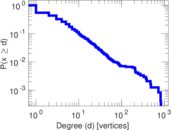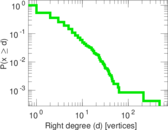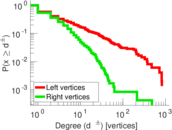### Lorenz curve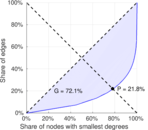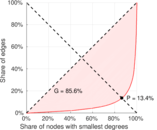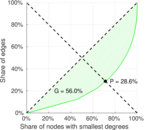### Spectral distribution of the adjacency matrix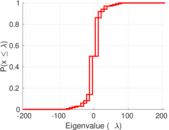### Spectral distribution of the normalized adjacency matrix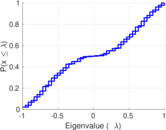### Spectral distribution of the Laplacian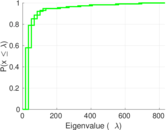### Spectral graph drawing based on the adjacency matrix### Spectral graph drawing based on the Laplacian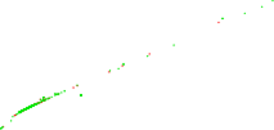### Spectral graph drawing based on the normalized adjacency matrix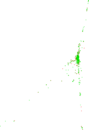### Degree assortativity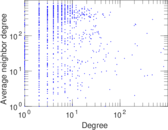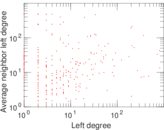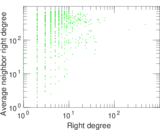### Zipf plot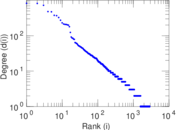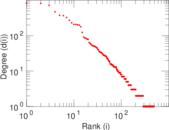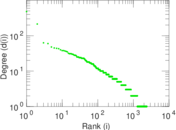### Hop distribution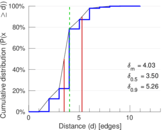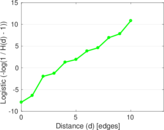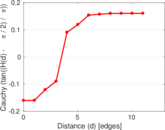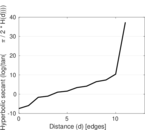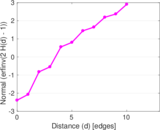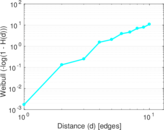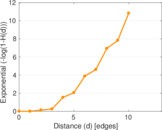### Double Laplacian graph drawing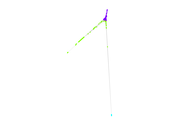### Delaunay graph drawing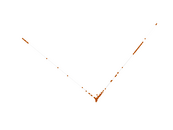### Edge weight/multiplicity distribution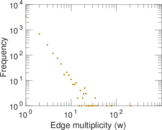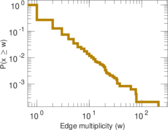### Temporal distribution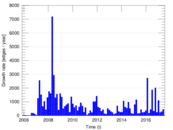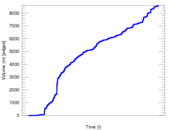### Temporal hop distribution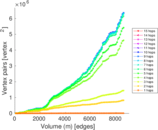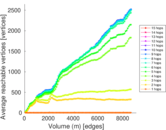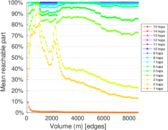### Diameter/density evolution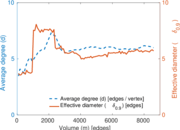### Matrix decompositions plots